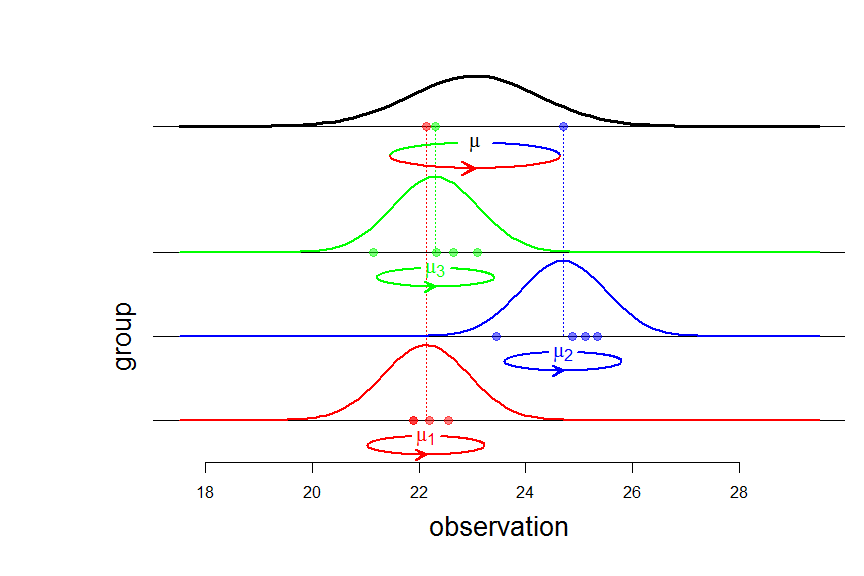# The balanced ANOVA model with random effects

Posted on July 29, 2017 by Stéphane Laurent
Tags: maths, statistics

$$\newcommand{\indic}{\mathbf{1}}$$ $$\newcommand{\perpoplus}{\overset{\perp}{\oplus}}$$ $$\newcommand{\RR}{\mathbb{R}}$$

## The balanced ANOVA model with random effects

The balanced ANOVA model is used to model a sample $$y=(y_{ij})$$ with a tabular structure: $y=\begin{pmatrix} y_{11} & \ldots & y_{1J} \\ \vdots & y_{ij} & \vdots \\ y_{I1} & \ldots & y_{IJ} \end{pmatrix},$ $$y_{ij}$$ denoting the $$j$$-th response in group $$i$$.

In the model with random effects, it is assumed that the observations $$y_{ij}$$ are generated in two steps. First, the group means $$\mu_i$$ are independently generated according to a Gaussian distribution $${\cal N}(\mu, \sigma^2_b)$$ where $$\mu$$ is the overall mean and $$\sigma^2_b$$ is the so-called between variance. Second, the responses $$y_{ij}$$, $$j =1,\ldots,J$$, for each group $$i$$, are independently distributed according to a Gaussian distribution $${\cal N}(\mu_i, \sigma^2_w)$$ with within variance $$\sigma^2_w$$ and mean $$\mu_i$$. Shortly, the model can be written: $\begin{cases} \mu_i \sim_{\text{iid}} {\cal N}(\mu, \sigma^2_b) & i=1, \ldots, I \\ (y_{ij} \mid \mu_i) \sim_{\text{iid}} {\cal N}(\mu_i, \sigma^2_w) & j = 1, \ldots, J \end{cases}.$An equivalent writing of this model, and from now on using capital letters for random variables, is $Y_{ij} = \mu + \sigma_bA_{i} + \sigma_wG_{ij},$ where all random variables $$A_i$$ and $$G_{ij}$$ are independent and $$\sim {\cal N}(0,1)$$.

## The three summary statistics $$\bar{Y}_{\bullet\bullet}$$, $$SS_b(Y)$$, $$SS_w(Y)$$

Using the tensor product language introduced in this article, the model can be written $Y = \mu({\bf 1}_I\otimes{\bf 1}_J) + \sigma_b A\otimes\indic_J +\sigma_wG, \qquad A \sim SN(\RR^I), \quad G \sim SN(\RR^I\otimes\RR^J).$

The overall mean $$\bar{Y}_{\bullet\bullet}$$ is given by the projection of $$Y$$ on the subspace $$[{\bf 1}_I]\otimes[{\bf 1}_J]$$:
$P_{[{\bf 1}_I]\otimes[{\bf 1}_J]} Y = \bar{Y}_{\bullet\bullet}({\bf 1}_I\otimes{\bf 1}_J).$

Then the variations $$Y_{ij}-\bar{Y}_{\bullet\bullet}$$ around the overall mean are given by the projection on the orthogonal complement $${\Bigl([\indic_I]\otimes[\indic_J]\Bigr)}^\perp$$. Knowing that $\RR^I \otimes \RR^J = \Bigl([\indic_I]\otimes[\indic_J]\Bigr) \perpoplus \Bigl([\indic_I]^\perp\otimes[\indic_J]\Bigr) \perpoplus \Bigl([\indic_I]\otimes[\indic_J]^\perp\Bigr) \perpoplus \Bigl([\indic_I]^\perp\otimes[\indic_J]^\perp\Bigr),$ one gets \begin{align} \underset{\text{'total'}}{\underbrace{ {\Bigl([\indic_I]\otimes[\indic_J]\Bigr)}^\perp} } & = \Bigl([\indic_I]^\perp\otimes[\indic_J]\Bigr) \perpoplus \Bigl([\indic_I]\otimes[\indic_J]^\perp\Bigr) \perpoplus \Bigl([\indic_I]^\perp\otimes[\indic_J]^\perp\Bigr) \\ & = \underset{\text{'between'}}{\underbrace{\Bigl([\indic_I]^\perp\otimes[\indic_J]\Bigr)}} \perpoplus \underset{\text{'within'}}{\underbrace{\Bigl(\RR^I\otimes[\indic_J]^\perp\Bigr)}}, \end{align} thereby yielding the decomposition of the variations: $P^\perp_{[\indic_I]\otimes[\indic_J]}Y = P_{[\indic_I]^\perp\otimes[\indic_J]}Y + P_{\RR^I\otimes[\indic_J]^\perp}Y,$ whose component formulae are:

• $${\bigl(P^\perp_{[\indic_I]\otimes[\indic_J]}Y\bigr)}_{ij}=Y_{ij}-\bar{Y}_{\bullet\bullet}$$

• $${\bigl(P_{[\indic_I]^\perp\otimes[\indic_J]}Y \bigr)}_{ij} = \bar{Y}_{i\bullet}-\bar{Y}_{\bullet\bullet}$$

• $${\bigl(P_{\RR^I\otimes[\indic_J]^\perp}Y\bigr)}_{ij} = Y_{ij}-\bar{Y}_{i\bullet}$$

Now we can see that the three summary statistics (overall mean, between sum of squares, within sum of squares) $\bar{Y}_{\bullet\bullet}, \quad SS_b(Y):={\Vert P_{[\indic_I]^\perp\otimes[\indic_J]}Y \Vert}^2, \quad SS_w(Y):={\Vert P_{\RR^I\otimes[\indic_J]^\perp}Y \Vert}^2,$ are independent random variables. Indeed, the overall mean $$\bar{Y}_{\bullet\bullet}$$ is given by \begin{align} P_{[{\bf 1}_I]\otimes[{\bf 1}_J]} Y &= \bar{Y}_{\bullet\bullet}({\bf 1}_I\otimes{\bf 1}_J) \\ & = \mu({\bf 1}_I\otimes{\bf 1}_J) + \sigma_b(P_{[{\bf 1}_I]}A)\otimes\indic_J+\sigma_wP_{[{\bf 1}_I]\otimes[{\bf 1}_J]}G, \end{align} the between variations are $P_{[\indic_I]^\perp\otimes[\indic_J]}Y = \sigma_b(P^\perp_{[\indic_I]} A)\otimes\indic_J + \sigma_w P_{[\indic_I]^\perp\otimes[\indic_J]} G,$ and the within variations are $P_{\RR^I\otimes[\indic_J]^\perp}Y = \sigma_wP_{\RR^I\otimes[\indic_J]^\perp} G.$ Independence follows from the independence between $$G$$ and $$A$$ and from the orthogonality between the ranges of the different projections.

It is easy to derive $$\bar{Y}_{\bullet\bullet} \sim {\cal N}\left(\mu, \frac{\sigma^2}{IJ}\right)$$ where $$\sigma^2=J\sigma^2_b+\sigma^2_w$$. It is also easy to get $$SS_w(Y) \sim \sigma^2_w\chi^2_{I(J-1)}$$ because of $P_{\RR^I\otimes[\indic_J]^\perp}Y = \sigma_wP_{\RR^I\otimes[\indic_J]^\perp}G.$ To derive the law of $$SS_b(Y)$$, note that \begin{align} P_{[\indic_I]^\perp\otimes[\indic_J]} G & = \begin{pmatrix} \bar{G}_{1\bullet} - \bar{G}_{\bullet\bullet} & \ldots & \bar{G}_{1\bullet} - \bar{G}_{\bullet\bullet} \\ \vdots & \vdots & \vdots \\ \bar{G}_{I\bullet} - \bar{G}_{\bullet\bullet} & \ldots & \bar{G}_{I\bullet} - \bar{G}_{\bullet\bullet} \end{pmatrix} \\ & = (P^\perp_{[\indic_I]}G_{\text{row}}) \otimes \indic_J \end{align} where $$G_{\text{row}} = (\bar{G}_{i\bullet})$$ is the vector of row means of $$G$$, and then one can write
$P_{[\indic_I]^\perp\otimes[\indic_J]} Y = \bigl(P^\perp_{[\indic_I]}(\sigma_b A + \sigma_w G_{\text{row}})\bigr) \otimes \indic_J.$ Now it is easy to see that the components of $$\sigma_b A + \sigma_w G_{\text{row}}$$ are $$\sim_{\text{iid}} {\cal N}(0, \sigma^2)$$, and consequently $$SS_b(Y) \sim \sigma^2\chi^2_{J-1}$$.

## Confidence interval for the overall mean

By our previous derivations, the statistic $\frac{\bar Y_{\bullet\bullet} - \mu}{\frac{1}{\sqrt{IJ}}\sqrt{\frac{SS_b(Y)}{I-1}}}$ has a Student $$t$$ distribution with $$I-1$$ degrees of freedom, wherefrom it is easy to get an exact confidence interval about the overall mean $$\mu$$.

Note that we would get exactly the same confidence interval if we were told only the group means $$\bar{Y}_{i\bullet}$$. This is the topic of another article.